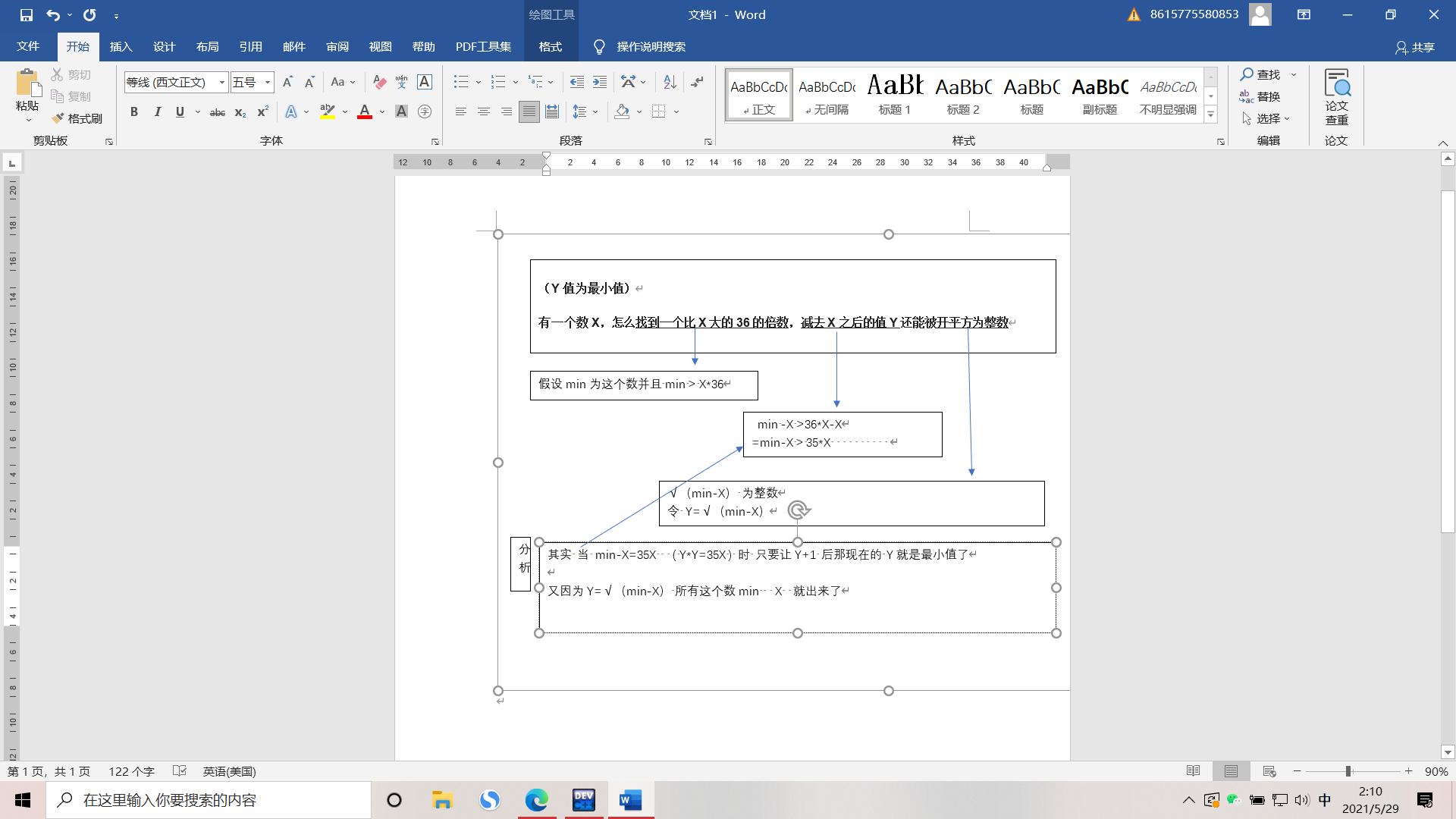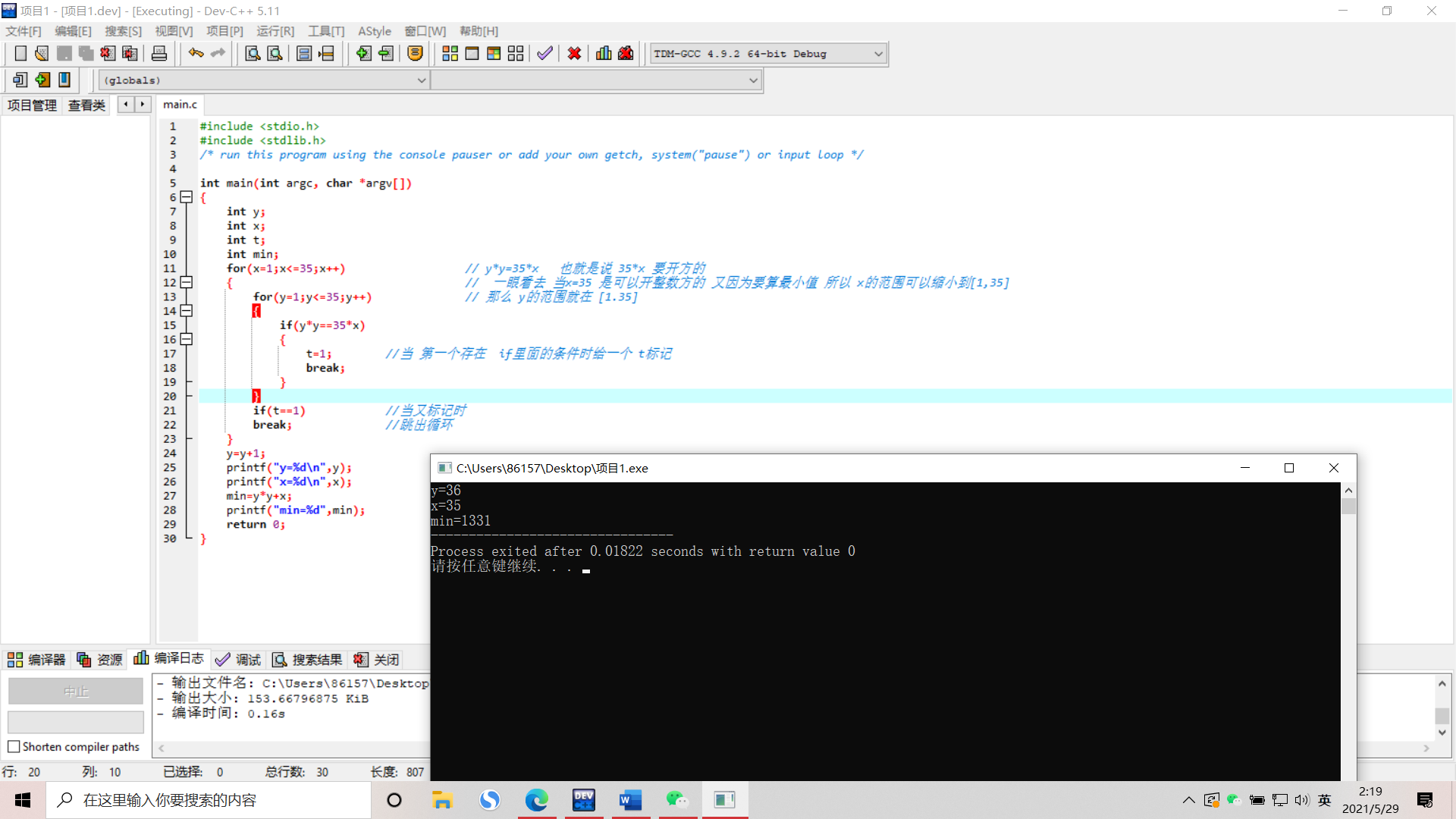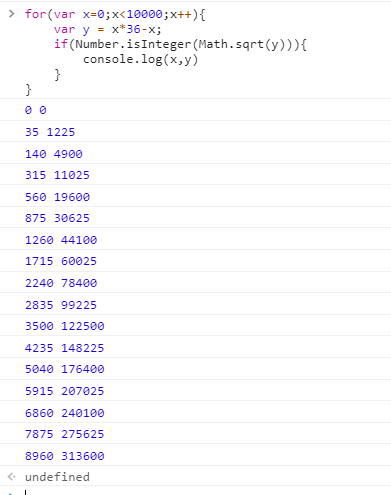2021-05-23 15:05

# 有一个数X，怎么找到一个比X大的36的倍数，减去X之后的值Y还能被开平方为整数（Y值为最小值）

• 点赞
• 写回答
• 关注问题
• 收藏
• 邀请回答

#### 2条回答默认 最新

•xiexiexiexieqing 2021-05-29 02:21``````#include <stdio.h>
#include <stdlib.h>
/* run this program using the console pauser or add your own getch, system("pause") or input loop */

int main(int argc, char *argv[])
{
int y;
int x;
int t;
int min;
for(x=1;x<=35;x++)            // y*y=35*x   也就是说 35*x 要开方的
{     //  一眼看去 当x=35 是可以开整数方的 又因为要算最小值 所以 x的范围可以缩小到[1,35]
for(y=1;y<=35;y++)     // 那么 y的范围就在 [1.35]
{
if(y*y==35*x)
{
t=1;        //当 第一个存在  if里面的条件时给一个 t标记
break;
}
}
if(t==1)            //当又标记时
break;              //跳出循环
}
y=y+1;
printf("y=%d\n",y);
printf("x=%d\n",x);
min=y*y+x;
printf("min=%d",min);
return 0;
}``````点赞 评论
•风雪一更 2021-06-22 11:39

10000以内的x有这些点赞 评论